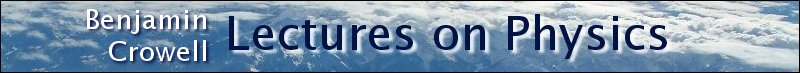Lectures on Physics has been derived from Benjamin Crowell's Light and Matter series of free introductory textbooks on physics. See the editorial for more information....# Homework problems(a) Realistic picture of a neuron. (b) Simplified diagram of one segment of the tail (axon).

The figure shows a neuron, which is the type of cell your nerves are made of. Neurons serve to transmit sensory information to the brain, and commands from the brain to the muscles. All this data is transmitted electrically, but even when the cell is resting and not transmitting any information, there is a layer of negative electrical charge on the inside of the cell membrane, and a layer of positive charge just outside it. This charge is in the form of various ions dissolved in the interior and exterior fluids. Why would the negative charge remain plastered against the inside surface of the membrane, and likewise why doesn't the positive charge wander away from the outside surface?

Use the nutritional information on some packaged food to make an order-of-magnitude estimate of the amount of chemical energy stored in one atom of food, in units of joules. Assume that a typical atom has a mass of 10 -26 kg. This constitutes a rough estimate of the amounts of energy there are on the atomic scale. [See chapter 1 of book 1, Newtonian Physics, for help on how to do order-of-magnitude estimates. Note that a nutritional "calorie" is really a kilocalorie.]
Recall that the potential energy of two gravitationally interacting spheres is given by PE =-Gm 1m 2/r , where r is the center-to-center distance. What would be the analogous equation for two electrically interacting spheres? Justify your choice of a plus or minus sign on physical grounds, considering attraction and repulsion. (b) Use this expression to estimate the energy required to pull apart a raisin-cookie atom of the one-electron type, assuming a radius of 10 -10 m. (c) Compare this with the result of the previous problem.
A neon light consists of a long glass tube full of neon, with metal caps on the ends. Positive charge is placed on one end of the tube, and negative charge on the other. The electric forces generated can be strong enough to strip electrons off of a certain number of neon atoms. Assume for simplicity that only one electron is ever stripped off of any neon atom. When an electron is stripped off of an atom, both the electron and the neon atom (now an ion) have electric charge, and they are accelerated by the forces exerted by the charged ends of the tube. (They do not feel any significant forces from the other ions and electrons within the tube, because only a tiny minority of neon atoms ever gets ionized.) Light is finally produced when ions are reunited with electrons. Compare the magnitudes and directions of the accelerations of the electrons and ions.
If you put two hydrogen atoms near each other, they will feel an attractive force, and they will pull together to form a molecule. (Molecules consisting of two hydrogen atoms are the normal form of hydrogen gas.) Why do they feel a force if they are near each other, since each is electrically neutral? Shouldn't the attractive and repulsive forces all cancel out exactly? Use the raisin cookie model. (Students who have taken chemistry often try to use fancier models to explain this, but if you can't explain it using a simple model, you probably don't understand the fancy model as well as you thought you did!)The figure shows one layer of the three-dimensional structure of a salt crystal. The atoms extend much farther off in all directions, but only a six-by-six square is shown here. The larger circles are the chlorine ions, which have charges of -e. The smaller circles are sodium ions, with charges of +e. The center-to-center distance between neighboring ions is about 0.3 nm. Real crystals are never perfect, and the crystal shown here has two defects: a missing atom at one location, and an extra lithium atom, shown as a grey circle, inserted in one of the small gaps. If the lithium atom has a charge of +e, what is the direction and magnitude of the total force on it? Assume there are no other defects nearby in the crystal besides the two shown here. [Hints: The force on the lithium ion is the vector sum of all the forces of all the quadrillions of sodium and chlorine atoms, which would obviously be too laborious to calculate. Nearly all of these forces, however, are canceled by a force from an ion on the opposite side of the lithium.]

The Earth and Moon are bound together by gravity. If, instead, the force of attraction were the result of each having a charge of the same magnitude but opposite in sign, find the quantity of charge that would have to be placed on each to produce the required force.In the semifinals of an electrostatic croquet tournament, Jessica hits her positively charged ball, sending it across the playing field, rolling to the left along the x axis. It is repelled by two other positive charges. These two equal charges are fixed on the y axis at the locations shown in the figure. (a) Express the force on the ball in terms of the ball's position, x. (b) At what value of x does the ball experience the greatest deceleration? Express you answer in terms of b. [Based on a problem by Halliday and Resnick.]

Last Update: 2009-06-21